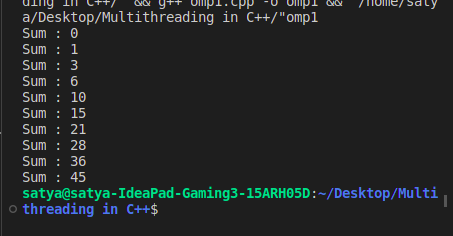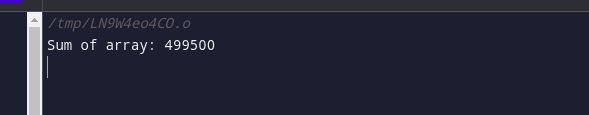×

Search anything:

# Multithreaded for loop in C++

#### C++Get this book -> Problems on Array: For Interviews and Competitive Programming

In this article at OpenGenus, we will learn about Multithreaded for loops in C++, Multithreading concept helps us to run many programs simultaneously at a time, resulting in making the system faster and multi-tasking.Before diving into the topic we should know Why do we Multithread loops?.

The loops are divided into parts and all parts run simulataneously, as a result program will be executed faster.

### Table Content:

• OpenMP pragma
• Multithreading Loop in C++ using OpenMP

Running multiple threaads at a time is reffered to as multithreading. The threads are executed parallaly i.e. more than one thread in being executed simulataneously.

There are different types/cases of parallelization are:

• Data Parallelization
Threads of same operations are executed with different data sets at a time.

• Model Parallelization
A single large model is being spitted into multiple threads and each thread commputes different part of the model and at last all outputs from the threads are combined together to get the final result.

• Hybrid Parallelization
This is cobination of other types of parallelization.

• Shared Parallelization
In this multiple threads running parallaly share a common memory space which help them to communicate and co-ordinate their activities more efficiently.

• Distributed Parallelization
In this multiple threads are spread across multiple systems and they communicate with each other to complete the task. Each system is called a node.

## OpenMP pragma

Before advancing further having a idea about pragmas and different OMP pragmas, will help us learn the implementation quicker and in more depth.

#pragma is a compiler directive used to give additional information(special features) to the compiler in c/c++ language.
OpenMP pragmas are use to create and set up a parallel region for multiple threads to run.
More about OMP pragma can be found at pragma omp parallel and pragma omp parallel for

## Multithreading for loop in C++ using OpenMP

OpenMP(Open Multi-Processing) is a library used for loop level multithreading works. It is one of the most simplest to create multiple threads for loops in C,C++ and Fortan. You cal leran about OpenMP and it's installation process from Install OpenMP.

Below there is a simple implementation of OpenMP to calculate the sum of the elements in array using multi-threading concept

``````#include <iostream>
#include <omp.h>
using namespace std;
``````

the omp.h header file contains all the required programs to use OpemMP

``````int main()
{
const int N = 10;
int a[N];

// initialize array
for (int i = 0; i < N; i++)
{
a[i] = i;
}

int sum = 0
#pragma omp parallel for reduction(+ \
: sum)
for (int i = 0; i < N; i++)
{
sum += a[i];
cout << "Sum : " << sum << endl;
}

return 0;
}
``````

the #pragma omp parallel for reduction(+ \ : sum) directive tells the compiler to parallelize the following loop. The + \ : sum this instructions means the process will add the result to sum variable and at last add them all to the get the final answer

### Output:To implement this approach the std::thread class is to be used.This class will allow to create and manage threads in our code.

Below there is a simple implementation of std::tread class to calculate the sum of the elements in array using multi-threading concept

``````#include <iostream>
#include <vector>
using namespace std;
``````

``````// Function to compute the sum of an array
int sum(const vector<int> &arr, int start, int end)
{
int result = 0;
for (int i = start; i < end; ++i)
{
result += arr[i];
}
return result;
}
``````
``````int main()
{
const int array_size = 1000;
vector<int> arr(array_size);
``````

initialising the number of threads and array size. Also declared a vector to store the answers from the threads.

``````    // Initialize the array with some values
for (int i = 0; i < array_size; ++i)
{
arr[i] = i;
}

// Create a vector of threads

// Divide the array into equal-sized chunks and create a thread for each chunk
int chunk_size = array_size / num_threads;
for (int i = 0; i < num_threads; ++i)
{
int start = i * chunk_size;
int end = (i + 1) * chunk_size;
{ results[i] = sum(arr, start, end); });
}
``````

``````    // Wait for all threads to finish
{
}

// Compute the final sum
int final_sum = 0;
for (int i = 0; i < num_threads; ++i)
{
final_sum += results[i];
}

// Print the final sum
cout << "Sum of array: " << final_sum << endl;

return 0;
}
``````

### Output:Parallelizing the loops are benifitial when they are CPU bounded resulting in spending less time and high performance of the CPU.